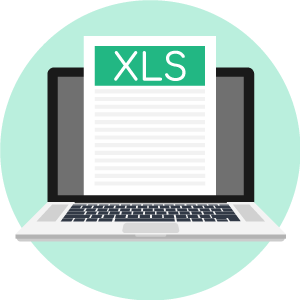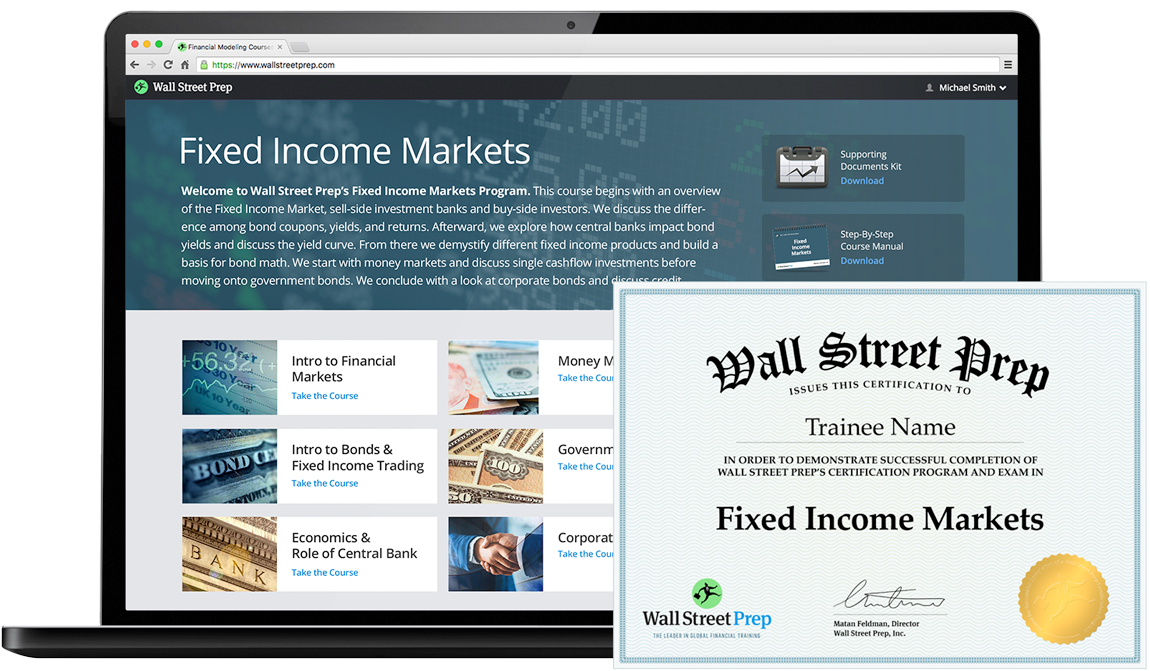# Coupon Rate

Understand the Coupon Rate Concept• What is the definition of the coupon rate?
• Which formula calculates the coupon rate?
• How can the annual coupon payment be calculated using the coupon rate?
• Are most bonds priced as fixed or variable coupon rates?

## Coupon Rate Definition

The coupon rate, or “nominal yield,” is the rate of interest paid to a bondholder by the issuer of the debt.

The coupon rate on a bond issuance is used to calculate the dollar amount of coupon payments paid, i.e. the periodic interest payments by the issuer to bondholders.

Coupons are the periodic interest payments received by bondholders from the original date of a bond issuance until the date of maturity – which is determined by the coupon rate as part of the bond issuance agreement.

• Coupon Payment (C): The dollar amount of the periodic interest paid to a bondholder by the issuer.
• Coupon Rate (%): The par value is multiplied by the coupon rate to calculate the interest on the bond.

Bonds are a form of raising capital for government entities and corporates alike, often for meeting liquidity needs and/or funding day-to-day operations.

As part of the bond indenture (i.e. the lending agreement), the issuer has a contractual obligation to service periodic coupon payments to the bondholder.

Originally, the name “coupon” comes from when coupons were physically attached to the documentation as a formal certificate noting the amounts and the dates of when interest payments come due.

## Coupon Rate Formula

The amount of interest due is based on the original principal of the bond (or initial investment), which will be stated on the bond security certificate.

Until the date of maturity, each periodic coupon payment must be paid on time, per the lending schedule.

At maturity, the face value (i.e. the par value) of the bond is returned in full to the bondholder, marking the end of the coupon payments.

### Bond Coupon Rate Formula

The formula for the coupon rate consists of dividing the annual coupon payment by the par value of the bond.

• Coupon Rate = Annual Coupon / Par Value of Bond

For example, if the coupon rate on a bond is 6% on a \$100k bond, the coupon payment comes out to \$6k per year.

• Par Value = \$100,000
• Coupon Rate = 6%
• Annual Coupon = \$100,000 x 6% = \$6,000

Since most bonds pay interest semi-annually, the bondholder receives two separate coupon payments of \$3k each year for as long as the bond is still outstanding.

• Number of Periods (N) = 2
• Coupon Payment = \$6,000 / 2 = \$3,000

## Bond Coupon Rate Calculation Steps

The coupon rate on a bond issuance can be calculated using the following four-step process:

1. First, the face (par) value of the bonds must be found in the financing documents (e.g. bond certificate).
2. Next, the number of periodic payments per year expected to be made by the issuer should be found using the same document source as in the previous step.
3. Then, the total annual coupon can be calculated by multiplying the periodic payment amount by the number of periodic payments per yer as noted in the bond indenture.
4. Lastly, the coupon rate is calculated by dividing the annual coupon payment by the face (par) value of the bond – which must be multiplied by 100%.

## Fixed vs Variable Coupon Bonds

The stated interest rate can be structured in the form of either:

• Fixed-Coupon Rate: In a fixed interest rate, the coupon rate remains fixed throughout the entire term of the bond, making the coupons more predictable.
• Variable Coupon Rate: In a floating interest rate, the coupon rate is based on an underlying benchmark (e.g. LIBOR, SOFR) – thus, the coupon payment fluctuates based on the benchmark.

Generally, for most fixed income instruments such as corporate bonds and municipal bonds, the fixed-coupon rate tends to be far more common.

## Coupon Rate Calculator – Excel Template

We’ll now move to a modeling exercise, which you can access by filling out the form below.Submitting ...

## Bond Coupon Rate Example Calculation

In our illustrative scenario, we’ll calculate the coupon rate on a \$1 million bond issuance.

###### Bond Assumptions
• Par Value of Bond (FV): \$1,000,000
• Frequency of Coupon (n): 2
• Coupon Payment (C): \$25,000

The frequency of the coupon payment is 2x per year, so the bond pays coupons semi-annually.

If we multiply the coupon payment by the frequency of the coupon, we can calculate the annual coupon.

• Annual Coupon (C) = \$25,000 x 2
• Annual Coupon (C) = \$50,000

With all the inputs ready, we can now calculate the coupon rate by dividing the annual coupon by the par value of the bonds.

• Coupon Rate (%) = \$50,000 / \$1,000,000
• Coupon Rate (%) = 5%

Therefore, the bond is priced at a coupon rate of 5% on a \$1 million par value, resulting in two semi-annual payments of \$25,000 per year until the bond reaches maturity.Globally Recognized Certification Program

#### Get the Fixed Income Markets Certification (FIMC©)

Wall Street Prep's globally recognized certification program prepares trainees with the skills they need to succeed as a Fixed Income Trader on either the Buy Side or Sell Side.Inline FeedbacksLearn Online: Crash Course in Bonds

For those pursuing fixed income research, investments, sales and trading or investment banking.

X

The Wall Street Prep Quicklesson Series

7 Free Financial Modeling Lessons

Get instant access to video lessons taught by experienced investment bankers. Learn financial statement modeling, DCF, M&A, LBO, Comps and Excel shortcuts.